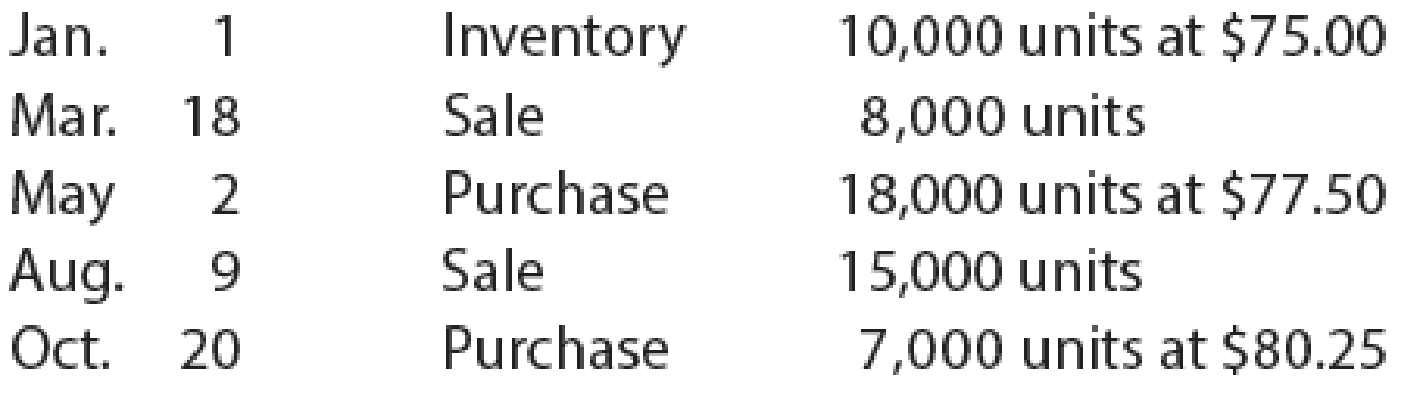# Weighted average cost flow method under perpetual inventory system The following units of a particular item were available for sale during the calendar year: The firm uses the weighted average cost method with a perpetual inventory system. Determine the cost of goods sold for each sale and the inventory balance after each sale. Present the data in the form illustrated in Exhibit 5.### Financial And Managerial Accounting

15th Edition
WARREN + 1 other
Publisher: Cengage Learning,
ISBN: 9781337902663

#### Solutions

Chapter
Section### Financial And Managerial Accounting

15th Edition
WARREN + 1 other
Publisher: Cengage Learning,
ISBN: 9781337902663
Chapter 6, Problem 9E
Textbook Problem
1 views

## Weighted average cost flow method under perpetual inventory systemThe following units of a particular item were available for sale during the calendar year:The firm uses the weighted average cost method with a perpetual inventory system. Determine the cost of goods sold for each sale and the inventory balance after each sale. Present the data in the form illustrated in Exhibit 5.

To determine

Determine the cost of goods sold and inventory balance after each sale using weighted average method as on December 31.

### Explanation of Solution

Perpetual Inventory System:

Perpetual Inventory System refers to the inventory system that maintains the detailed records of every inventory transactions related to purchases, and sales on a continuous basis. It shows the exact on-hand-inventory at any point of time.

Weighted -average cost method:

Under weighted average cost method, the company calculates a new average cost after every purchase is made. It is determined by dividing the cost of goods available for sale by the units on hand.

Calculate the cost of cost of goods sold and ending inventory using the weighted average method:

Table (1)

Working Notes (1):

Calculate the weighted average unit cost after June 30 purchases:

 Weighted average method Quantity Unit cost Total cost of inventory in hand 1,500 units $40$60,000 4,500 units $44$198,000 6,000 units \$ 258,000

Table (2)

Weighted average unit cost = Total cost of inventory in handT

### Still sussing out bartleby?

Check out a sample textbook solution.

See a sample solution

#### The Solution to Your Study Problems

Bartleby provides explanations to thousands of textbook problems written by our experts, many with advanced degrees!

Get Started